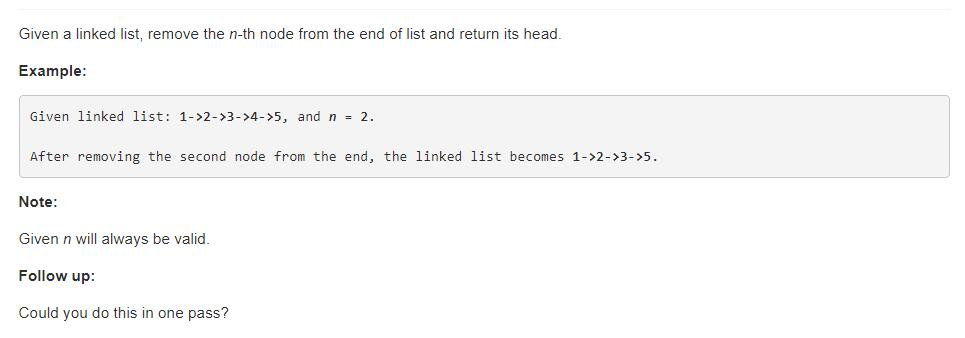# 题目描述（中等难度）# 解法一

public ListNode removeNthFromEnd(ListNode head, int n) {
int len = 0;
while (h != null) {
h = h.next;
len++;
}
//长度等于 1 ，再删除一个结点就为 null 了
if (len == 1) {
return null;
}

int rm_node_index = len - n;

//如果删除的是头结点
if (rm_node_index == 0) {
}

//找到被删除结点的前一个结点
for (int i = 0; i < rm_node_index - 1; i++) {
h = h.next;
}

//改变指向
h.next = h.next.next;
}


public ListNode removeNthFromEnd(ListNode head, int n) {
ListNode dummy = new ListNode(0);
int length  = 0;
while (first != null) {
length++;
first = first.next;
}
length -= n;
first = dummy;
while (length > 0) {
length--;
first = first.next;
}
first.next = first.next.next;
return dummy.next;
}


# 解法二 遍历一次链表

public ListNode removeNthFromEnd(ListNode head, int n) {
ListNode dummy = new ListNode(0);
ListNode first = dummy;
ListNode second = dummy;
//第一个指针先移动 n 步
for (int i = 1; i <= n + 1; i++) {
first = first.next;
}
//第一个指针到达终点停止遍历
while (first != null) {
first = first.next;
second = second.next;
}
second.next = second.next.next;
return dummy.next;
}


# 解法三

public ListNode removeNthFromEnd(ListNode head, int n) {
List<ListNode> l = new ArrayList<ListNode>();
int len = 0;
while (h != null) {
h = h.next;
len++;
}
if (len == 1) {
return null;
}
int remove = len - n;
if (remove == 0) {
}
//直接得到，不需要再遍历了
ListNode r = l.get(remove - 1);
r.next = r.next.next;
}